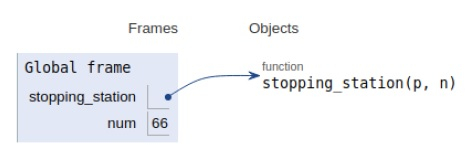# Python Program for Number of stopping station problem

Problem statement − We are given that there are 13 intermediate stations between two places A and B. We need to find the number of ways in which a train can be stopped at 2 intermediate stations, such that there are no consecutive stations?

Now let’s observe the solution in the implementation below −

## Example

Live Demo

# stop station
def stopping_station( p, n):
num = 1
dem = 1
s = p
# selecting specified position
while p != 1:
dem *= p
p-=1
t = n - s + 1
while t != (n-2 * s + 1):
num *= t
t-=1
if (n - s + 1) >= s:
return int(num/dem)
else:
# condition
return -1
# main
num = stopping_station(2, 13)
if num != -1:
print("No of stopping stations:",num)
else:
print("I'm Possible")

## Output

No of stopping stations: 66All the variables are declared in the local scope and their references are seen in the figure above.

## Conclusion

In this article, we have learned about how we can make a Python Program for the Number of stopping station problems.